# Calculus 2 : Series and Functions

## Example Questions

2 Next →

### Example Question #11 : Introduction To Series In Calculus

What do we mean when we say an infinite series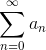converges?

The sequence partial sums of the sequence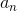, denoted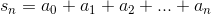converges as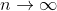.

The sequence partial sums of the sequence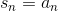converges as.

The sequence partial sums of the sequence, also denotedconverges as.

None of the other choices

The sequenceconverges as.

The sequence partial sums of the sequence, denotedconverges as.

Explanation:

This is the definition of a convergent infinite series.

### Example Question #12 : Introduction To Series In Calculus

What is the sum of the following geometric series: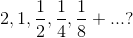Cannot be determined.Explanation:

Since this is a geometric series with a rate betweenand, we can use the following equation to find the sum: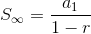, where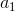is the starting number in the sequence, andis the common divisor between successive terms in the sequence.  In this sequence, to go from one number to the next, we multiply by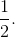Now, we plug everything into the equation: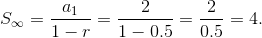### Example Question #13 : Introduction To Series In Calculus

Find the infinite sum of the following geometric series: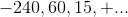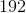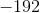Cannot be determined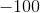Explanation:

Since this is a geometric series with a rate betweenand, we can use the following equation to find the sum:, whereis the starting number in the sequence, andis the common divisor between successive terms in the sequence.  In this sequence, to go from one number to the next, we multiply by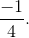Now, we plug everything into the equation: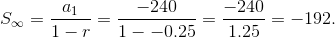### Example Question #14 : Introduction To Series In Calculus

Find the infinite sum of the following series: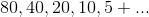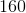Explanation:

For the sum of an infinite series, we have the following formula:, whereis the first term in the series andis the rate at which our series is changing between consecutive numbers in the series.  Plugging all of the relevant information for this series, we get: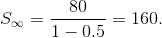### Example Question #15 : Introduction To Series In Calculus

Find the infinite sum of the following series: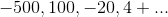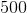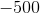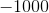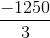Explanation:

For the sum of an infinite series, we have the following formula:, whereis the first term in the series andis the rate at which our series is changing between consecutive numbers in the series.  Plugging all of the relevant information for this series, we get: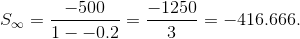### Example Question #16 : Introduction To Series In Calculus

A) Find a power series representation of the function,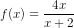B) Determine the power series radius of convergence.

A) Power Series for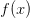,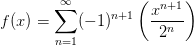2

A) Power Series for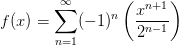A) Power Series for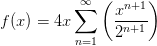A) Power Series for,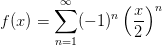1

A) Power Series for,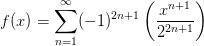1

A) Power Series forExplanation:This function can be easily written as a power series using the formula for a convergent geometric series.

____________________________________________________________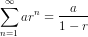For any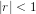____________________________________________________________

First let's make some modifications to the function so we can compare it to the form of a convergent geometric series: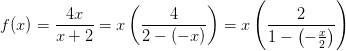Notice if we take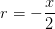and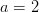we can writein the form,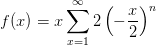We can find the radius of convergence by applying the condition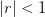.

_____________________________________________________________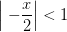Case 1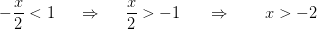Case 2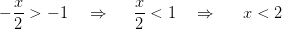Combing both cases gives the interval of convergence,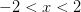Therefore the radius of convergence is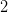____________________________________________________________

We can continue simplifying our most recent expression of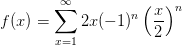### Example Question #17 : Introduction To Series In Calculus

Does the following series converge or diverge: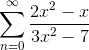Diverge

Converge

Cannot be determined with the given information.

Diverge

Explanation:

To test if this series diverges, before using a higher test, we may use the test for divergence.

The test for divergence informs that if the sequence does not approach 0 as n approaches infinity then the series diverges (NOTE: This only shows divergence, the converse is not true, that is, the test for divergence cannot be used to show convergence.).

We note that as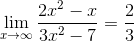,

this is derived from the fact that to find the limit as x approaches infinity of a function, one must first find the horizontal asymptote. Since this function is a rational expression with the highest power in both the numerator and denominator, the horizontal asymptote is equal to the quotient of the leading coefficients of both the numerator and denominator, which in this case is 2/3.

Since the limit as x tends to infinity of this series is a nonzero value, we may conclude that the series diverges by the Test for Divergence.

2 Next →